# data-scienceWhat is Big Data? Types, Characteristics and Examples

In this blog, we have discussed the idea of Big Data, which addresses the challenge of managing vast amounts of complex and diverse data. We discussed what constitutes Big Data, its types, characteristics, examples, use cases, the various technologies used, advantages, and the challenges faced by engineers working with Big Data.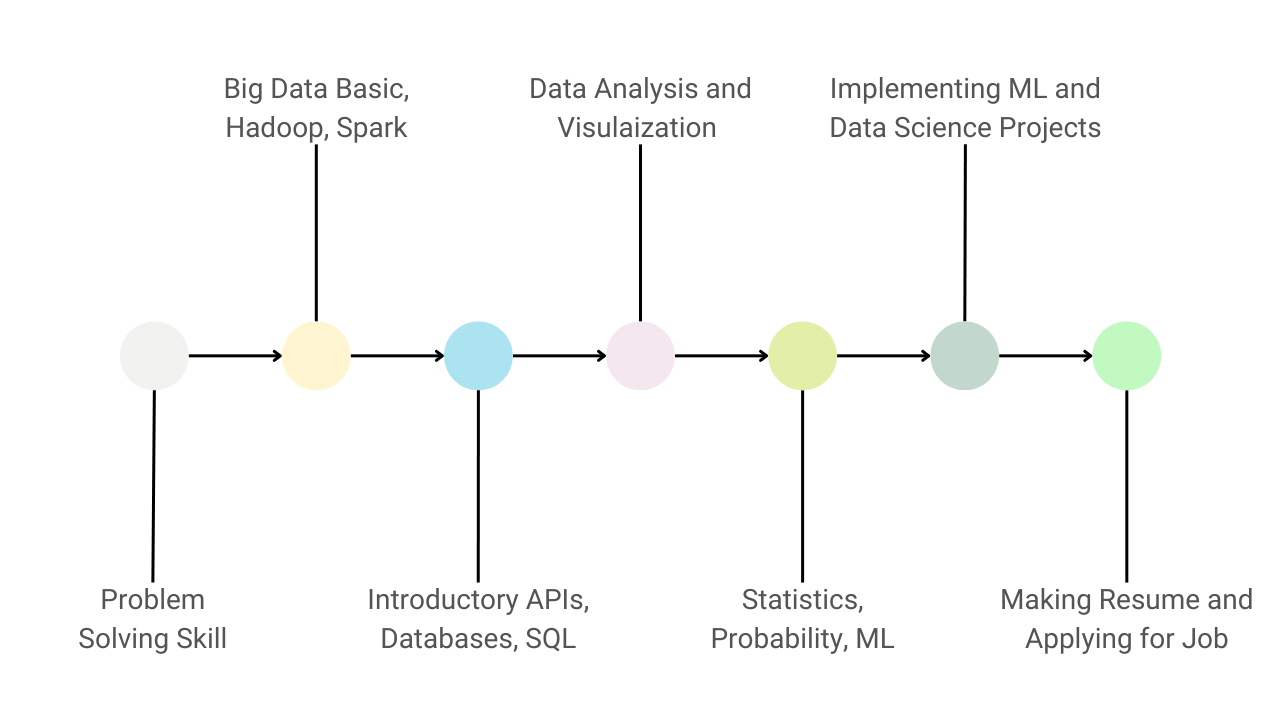Guide to Learn Data Science and Become a Data Scientist

Here are 7 key steps to master data science: 1) Learning Python 2) Understanding big data frameworks like Hadoop and PySpark 3) Learning concepts of APIs, databases and SQL 4) Hands-on experience in data analysis and visualization 5) Learning statistics, probability, and machine learning 6) Building data science projects 7) Making resume and applying for data scientist positions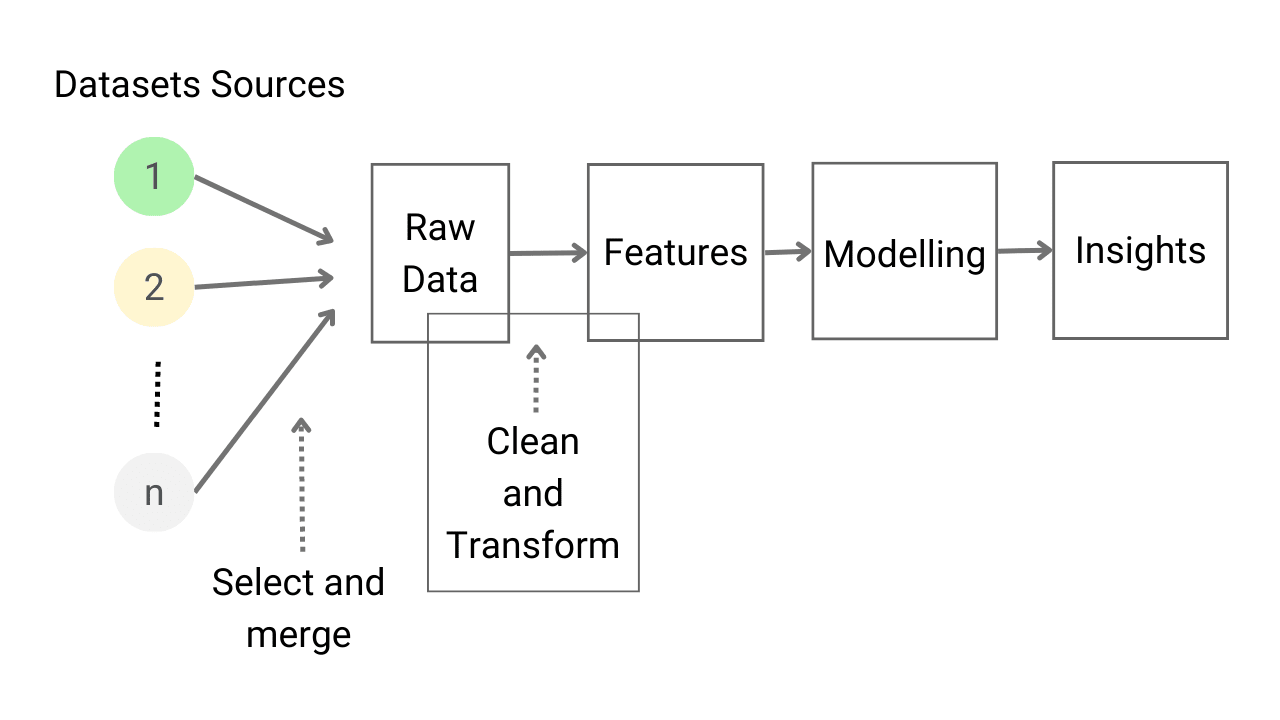Introduction to Feature Engineering for Machine Learning

Feature engineering is the process of selecting, correcting, and generating new features from existing attributes in a dataset to optimize the performance of machine learning models. The main steps involved are feature creation, transformation, extraction, and selection. To improve results, we can employ techniques such as imputation, transformation, scaling, and encoding, which will be discussed in this blog.Data Types and Variables in Python Programming

Python is the most preferred language for developing machine learning and data science applications. It has a large community support that can help debug the errors and resolve all the roadblocks appearing while developing any solution. In this blog, we have discussed various data types like integers, floats, boolean and strings along with their usage in Machine Learning and Data Science.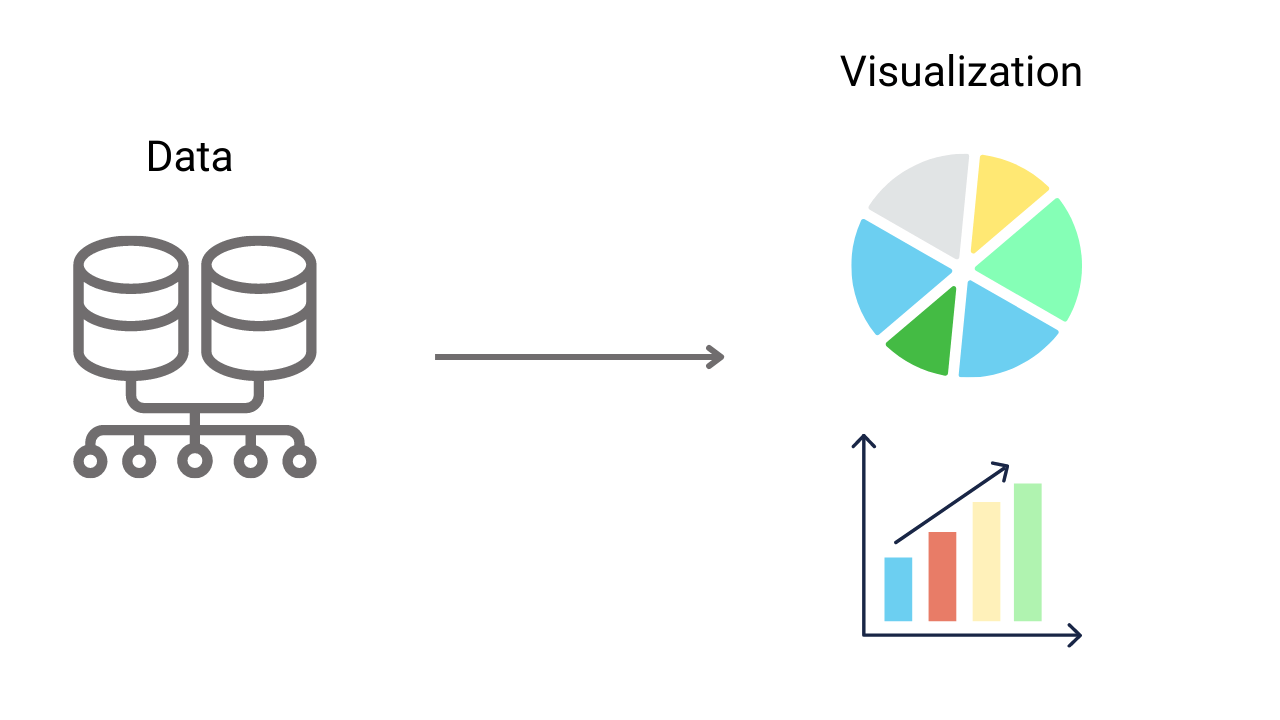Data Visualization Introduction In Data Science

Data Visualization is a technique for presenting information using visual elements, making it accessible and easy to comprehend. It plays a crucial role in all various stages of machine learning and data science. To be effective, data visualizations should be aesthetically simple, creative and informative. In this blog, we explore various processes and examples of data visualization.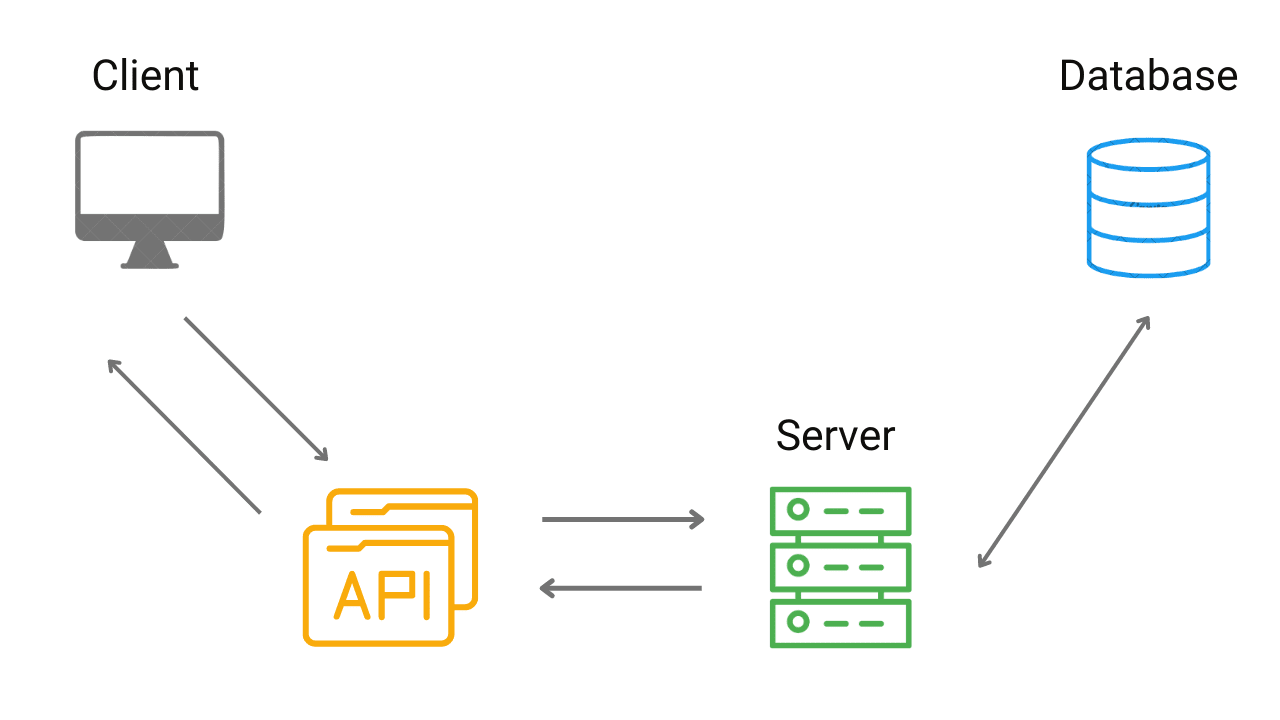Introduction to APIs for Data Science and Machine Learning

In machine learning and data science, API (Application Programming Interface) is a powerful tool that enables seamless communication and data sharing between applications and servers. APIs are mainly used for data gathering and model deployment In data science and ML. This blog provides a step-by-step explanation of how APIs work.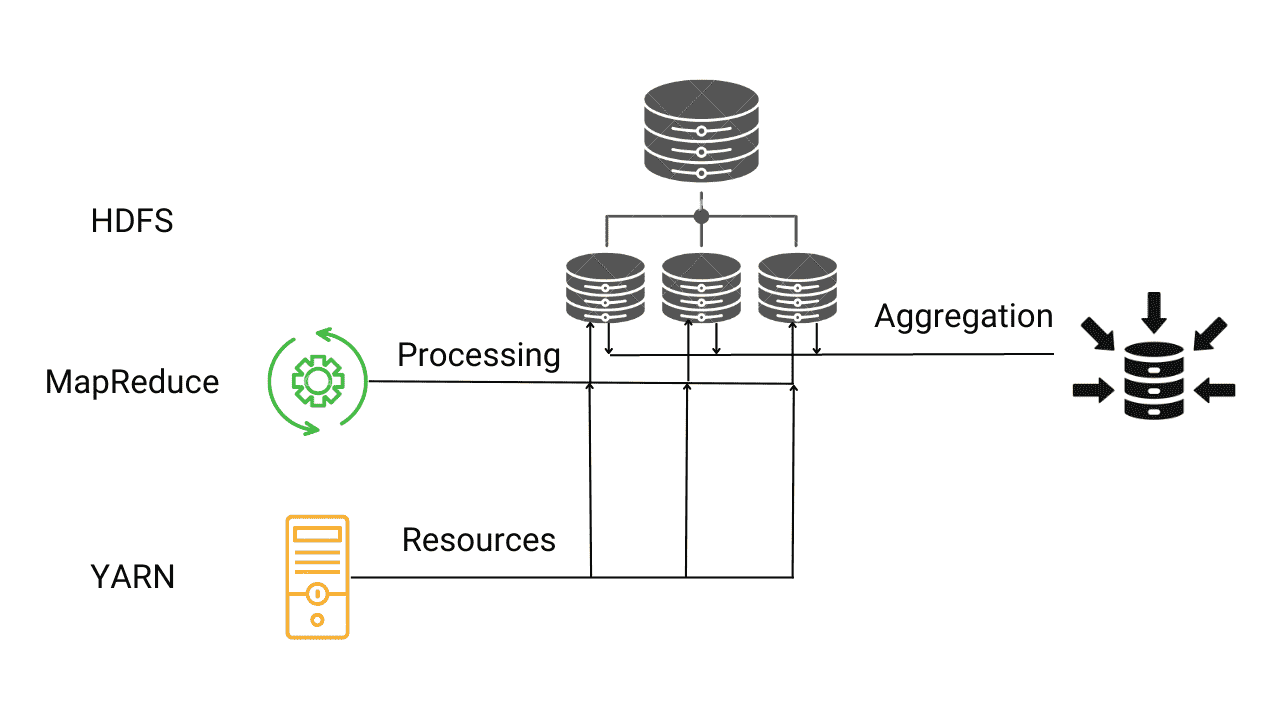Introduction to Hadoop Framework for Big Data

Hadoop is an open-source framework that addresses the analytical and operational needs of Big Data by overcoming the limitations of traditional data analysis methods. With support for highly scalable and fault-tolerant distributed file systems, it allows for parallel processing. It comprises four main components - HDFS, YARN, MapReduce, and Hadoop Common.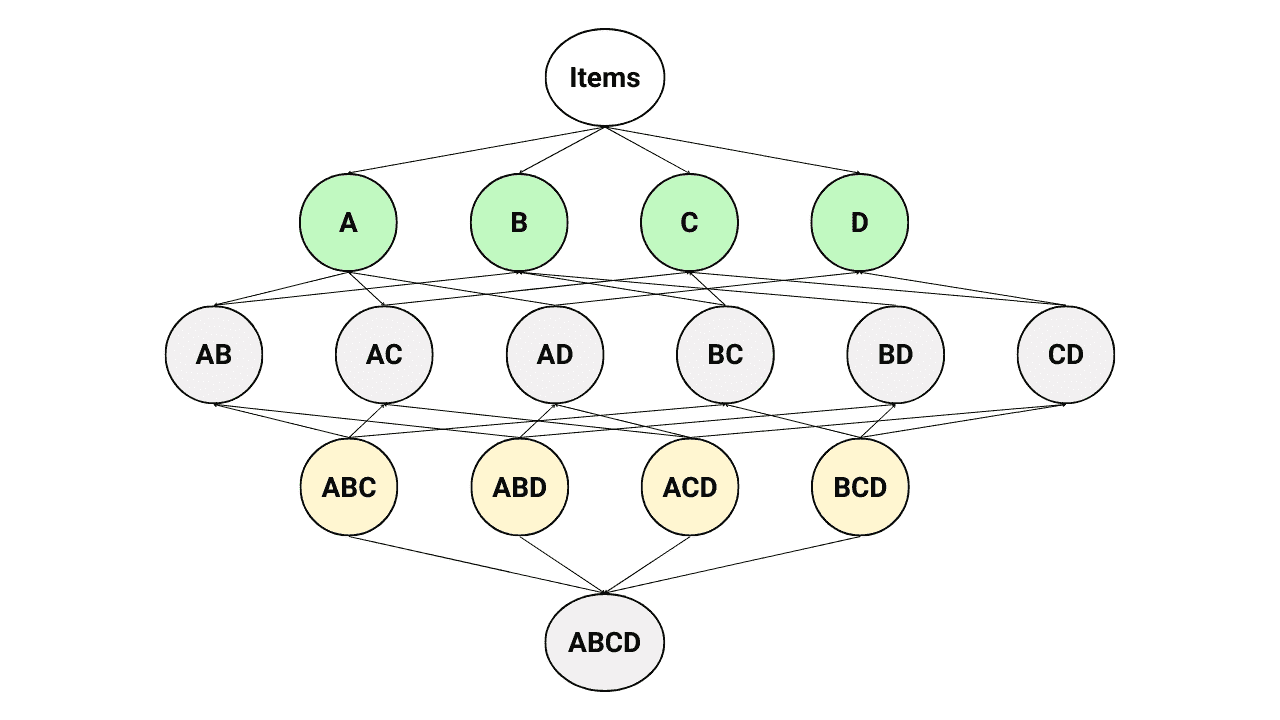What is Apriori Algorithm? Examples and Implementation

The Apriori Algorithm is a powerful tool in association rule mining that helps to uncover the relationships and associations among items. This technique is widely used by supermarkets and online shopping platforms to optimize product placement and offer discounts on bundled purchases. In this article, we have explained its step-by-step functioning and detailed implementation in Python.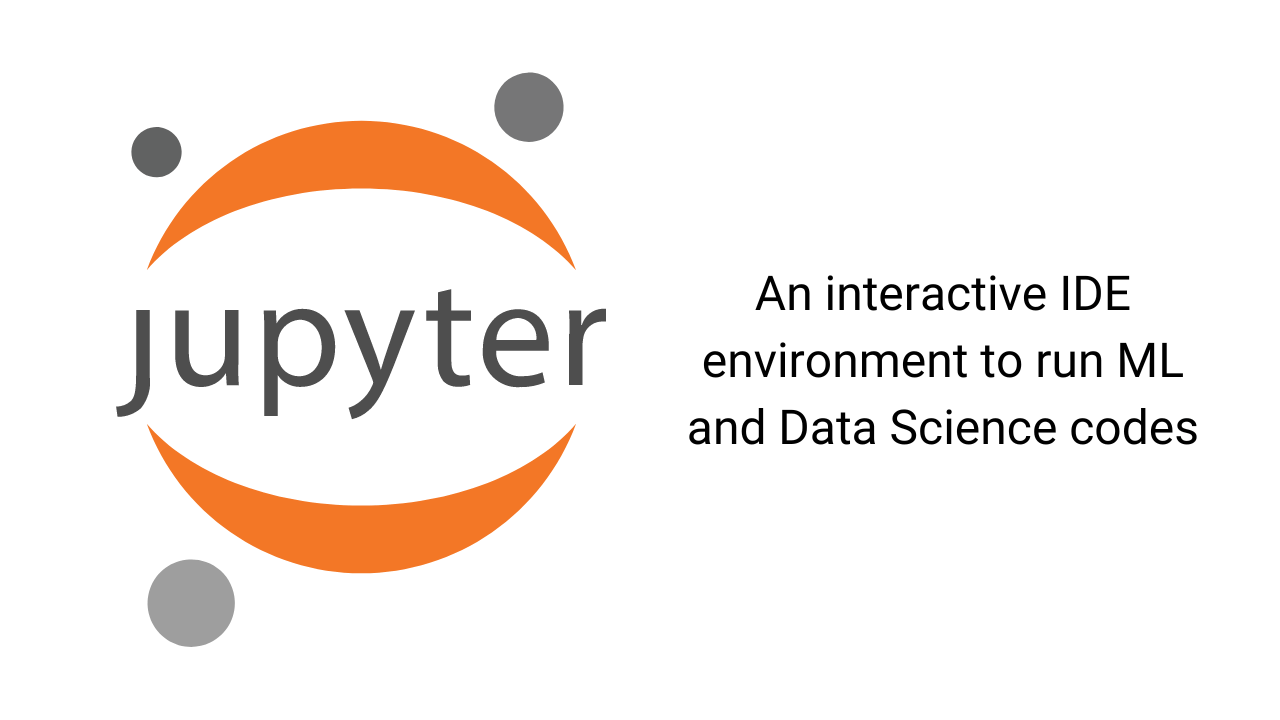Introduction to Jupyter Notebook

Jupyter Notebook is a popular open-source IDE for data science and machine learning practitioners. It supports three programming languages: Julia, Python, and R. In this guide, we cover the installation process for Jupyter Notebook, as well as provide an overview of the basic steps for starting the server, creating notebooks, executing cells, and working with kernels.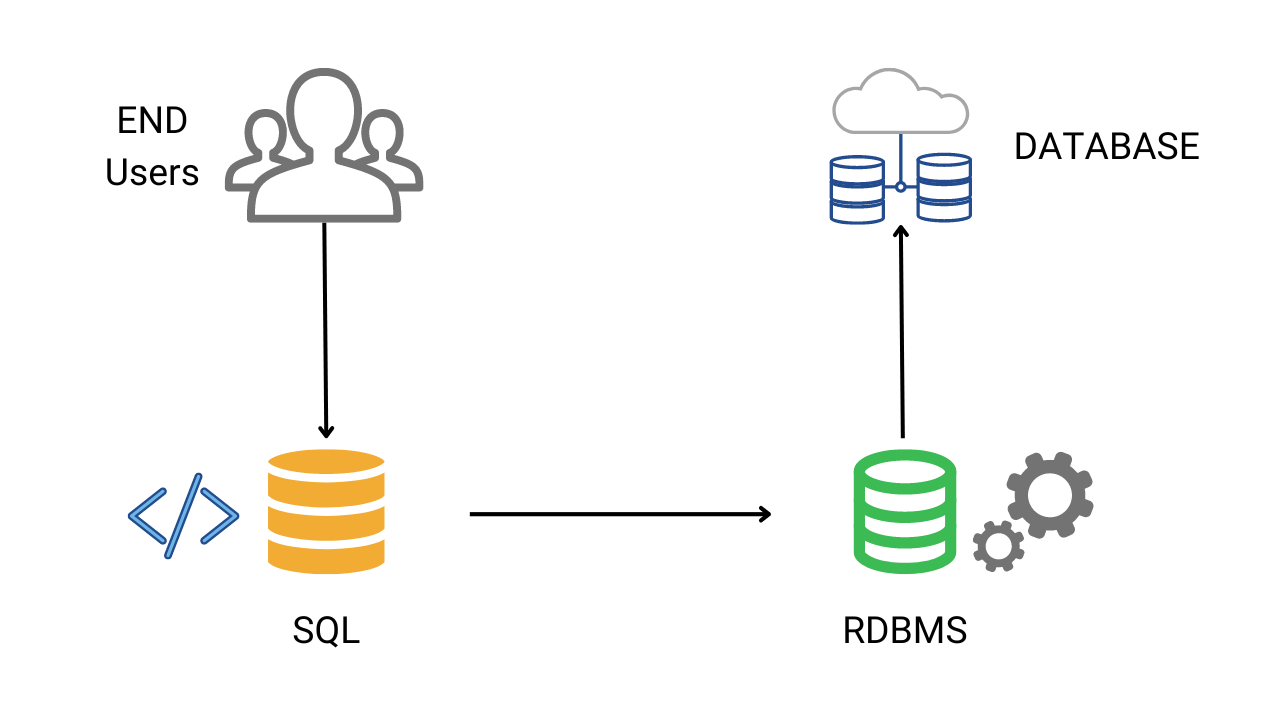Introduction to SQL for Data Science

Based on Reports, more than 40% of data science jobs require SQL as an essential skill. So, to analyze datasets effectively in data science, one should master RDBMS, data cleaning processes, and SQL commands. The major advantage of using SQL is that SQL queries can be executed easily in Python by establishing connections to the database.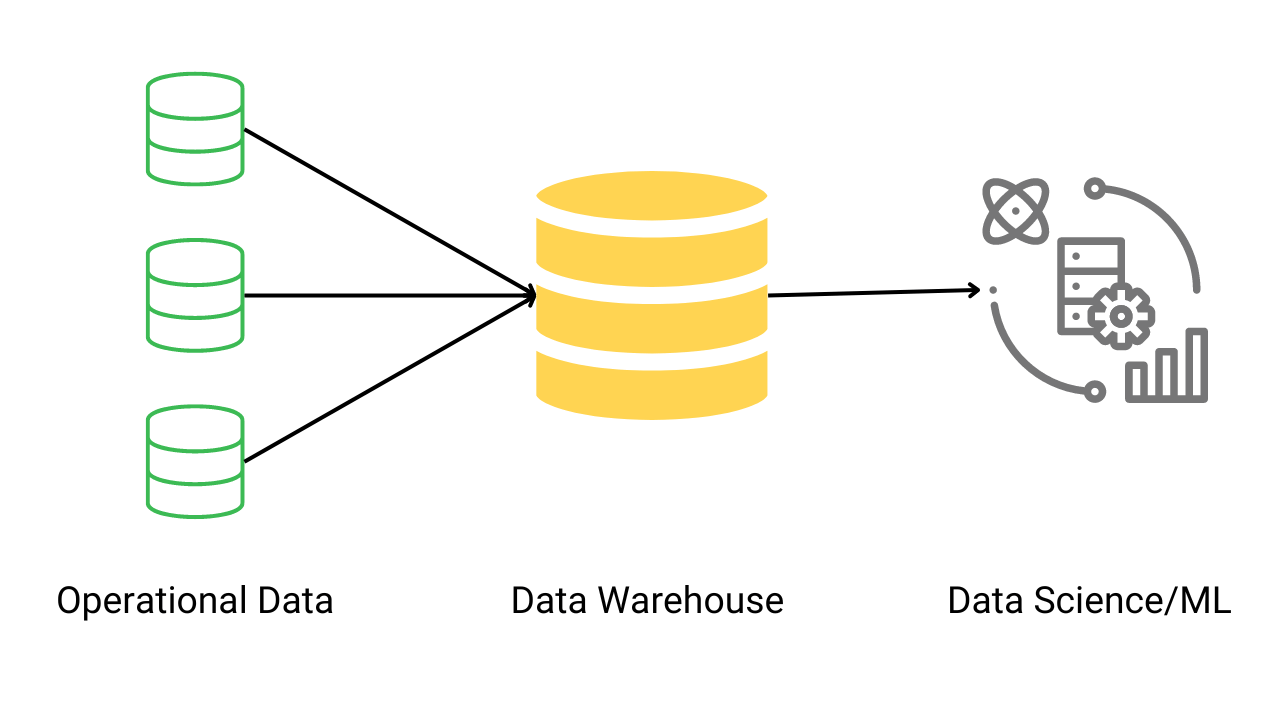Introduction to Databases for Data Science

In data science, databases play a crucial role in storing, managing, and scaling large amounts of data. This data is then analyzed to gain meaningful insights. In this blog, we will delve into the concept of databases and understand how data science relies on them, as well as their advantages.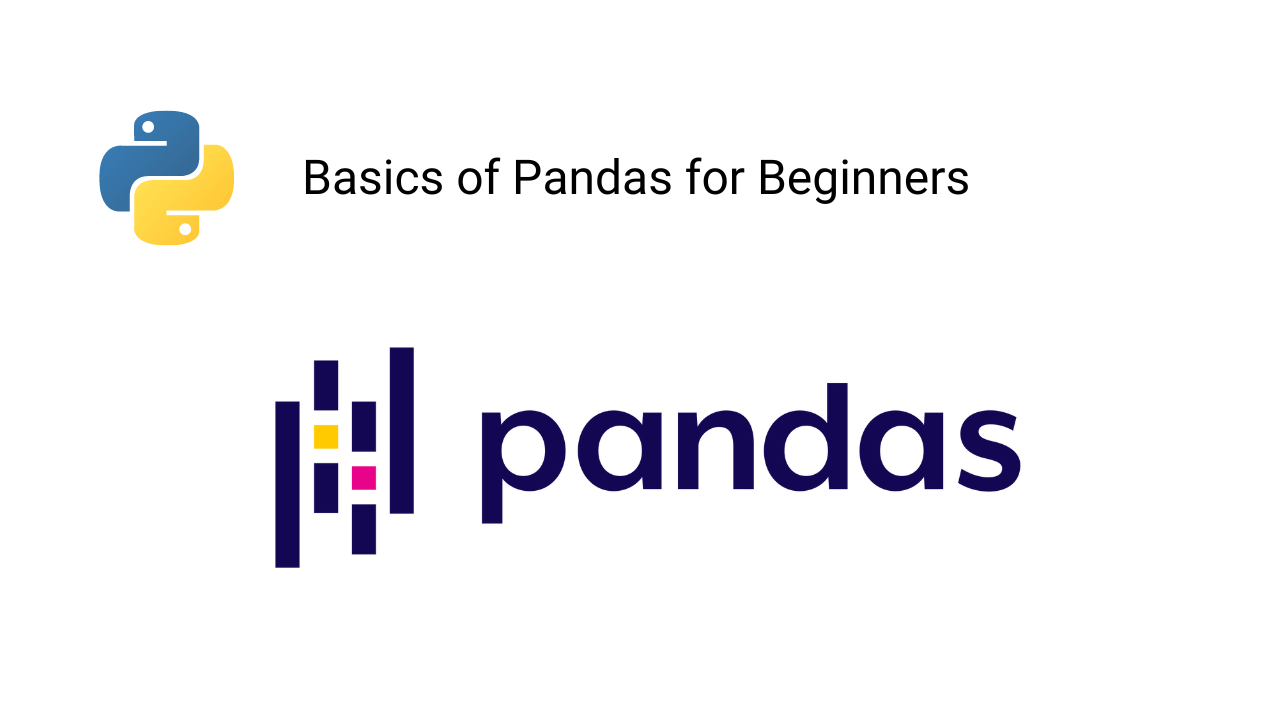Introduction to Pandas Library in Python

Pandas is a widely used Python library by data scientists for managing, processing, and analyzing data. This library offers various efficient tools and functions to streamline data manipulation and analysis. In this blog, we will guide you through the installation process and provide an overview of the frequently used basic Pandas functions in machine learning projects.Introduction to NumPy in Python

Numpy is one of the most essential Python libraries for building machine learning and data science applications. Its ability to perform parallel computing and execute certain functions using C programming makes it lightning-fast. In this blog, we will cover the basics of the Numpy library, including installation and the most commonly used functions for executing mathematical operations.What is Data Science? Introduction and Importance

These days, companies are collecting huge amounts of data, but the question is: what is the purpose of this data? This is where data science comes into play. Data science is a field that extracts, processes, analyzes, and interprets data to derive various insights from it. In this blog, we will discuss the importance of data science and various key concepts.Feature Selection Techniques in ML

The best machine learninmodel would have the lowest number of features involved in the analysis keeping the performance high. Therefore, determining the relevant features for the model building phase is necessary. In this session, we will see some feature selection methods and discuss the pros and cons of each.Drug Discovery using Machine Learning

Machine Learning and Data Science have become vital in developing life-saving applications, such as drug discovery. Using these ML technologies, researchers can reduce the time needed to create new medicines for known and unknown diseases. In this blog post, we provide a step-by-step guide to building an application that addresses the drug discovery problem using Machine Learning in Python. To solve this regression problem, we utilized the XGBoost regressor model, which is a popular CART algorithm in the ML community.Music Recommendation System Using Machine Learning

Learn to build a music recommendation system using the k-means algorithm. We will use the audio features from the million song data and cluster them based on their similarities. In this blog, we will be discussing these topics: 1) Methods to build a recommendation system for songs 2) Step-wise implementation 3) Ordering songs for the recommendation, etc.Image Compression Using Machine Learning

In this blog, we will build an image data compressor using an unsupervised learning technique called Principal Component Analysis (PCA). We will be discussing image types and quantization, step-by-step Python code implementation for image compression using PCA, and techniques to optimize the tradeoff between compression and the number of components to retain in an image.Conditions and Branching in Python

We sometimes need to execute specific instructions only when some conditions are true. If not, then we will perform a different set of instructions. In this blog, we have discussed: 1) Various comparison operations in Python. 2) What are conditions in python? 3) What is branching? 3) How do we use logical operations to combine the two conditions? etc.Loop in Python Programming

Loops are the set of instructions that needs to be executed repeatedly until a defined condition is satisfied. In this blog, we have discussed the range function in python working of a loop and the most popular loops: for and while loop in python. The use of continue and break statements in loop increase their usability in Python and building ML Applications.Functions in Python Programming

Functions are a set of instructions grouped in a block and get executed only when it is called inside our program. In python programming, functions follow specific syntaxes to ensure their validity. In this blog, we have discussed: What are functions in python? How to create and call functions? Various types of function arguments, The anonymous function and most used python in-built functions in ML and Data Science projects.Introduction to Seaborn in Python

Seaborn is an open-source library built over Matplotlib and makes plots more appealing and understandable. It works excellently with data frames and pandas libraries. In this blog, we have discussed: 1) Advantages of Seaborn over Matplotlib library, 2) Installation process of Seaborn in Python 3) Various Data Plots using the Seaborn library.Matplotlib Library in Python

Matplotlib is one of Python's most effective visualization libraries for data visualization. It is an open-source library built over NumPy arrays. In this blog, we have discussed: 1) What is Matplotlib 2) Installation of Matplotlib using PIP 3) What is Pyplot in Matplotlib 4) The subplot in Matplotlib's pyplot module 5) Various plots using Matplotlib.Sets and Dictionaries in Pyhton Programming

In python, sets and dictionaries are unordered data structures frequently used in machine learning applications. In this blog, we have explained these concepts: 1) What is set in python? 2) Important operations on sets, 3) Conversion of lists into sets 4) What is dictionary in python? 5) Operations on dictionaries? 6) Comparison of sets and dictionaries.Sentiment Analysis using Naive Bayes

Sentiment analysis is a technique that comes under natural language processing(NLP) and is used to predict emotions reflected by a word or a group of words. Sentiment analysis is instrumental in brand monitoring, market research, social media monitoring, etc. This blog will discuss naive bayes to predict sentiments using their tweets.Tuples and Lists in Python Programming

Tuples and lists are two most popular python data structures used in Machine Learning and Data Science applications. They are also called compound data types because they can store a mixture of primitive data types like strings, ints, and floats. Tuples are static and immutable while lists are dynamic and mutable, but tuples are memory efficient as compared to lists. We will explore these data structures in detail in this blog.Python Date and Time

As data scientists, we should know how to handle the date-time data and the standard set of date-time operations we can apply to transform the raw data. Fortunately, we have date-time manipulation libraries specifically for this purpose. In this blog, we will talk about all basic date-time manipulations, explorations, transformations, and applications.t-SNE Algorithm in Machine Learning

t-SNE (t-distributed stochastic neighbor embedding) is an unsupervised non-linear dimensionality reduction algorithm used for exploring high-dimensional data. In this blog, we have discussed: What is t-SNE, difference between t-SNE and PCA in dimensionality reduction, step-wise working of t-SNE algorithm, t-SNE python implementation and Mathematical analysis of t-SNE algorithm.Applications of Regex in Data Science

In this blog, we will focus on applications of regex by implementing it to some tedious tasks that wouldn’t be possible without regular expressions. Some standard applications of regular expressions in data science: 1) Web scraping and data collection 2) Text preprocessing (NLP) 3) Pattern detection for IDs, e-mails, names 4) Date-time manipulationsEmployee Attrition Prediction using ML

In this blog, we have demonstrated data analysis of the company's attrition rate and built a machine learning model (logistic regression model) to predict it. We have explored some exciting patterns that lead to employee attrition. We will be using Kaggle's IBM HR analytics Employee Attrition and Performance dataset for this analysis.Regular Expression in ML (Python)

A regular expression is an expression that holds a defined search pattern to extract the pattern-specific strings. Today, regular expressions are available for almost every high-level programming language. As data scientists or machine learning engineers, we should know the fundamentals of regular expressions and when to use them.Anomaly Detection in Machine Learning

In machine learning, anomaly detection is a process of finding samples behaving abnormally compared to the majority of samples present in the dataset. Anomaly detection algorithms have important use-cases in data analytics and data science fields. For example, fraud analysts use anomaly detection algorithms to detect fraud transactions.K-Means Clustering Algorithm in Machine Learning

The K-means algorithm is one of the most widely used clustering algorithms in machine learning. It separates data into k distinct clusters based on predefined criteria. In this article, we discuss how the k-means algorithm works, provide a step-by-step implementation with Python code, cover popular methods for determining the optimal value of k in k-means, and introduce other important concepts.Univariate, Bivariate, and Multivariate Analysis

Exploratory data analysis can be classified as Univariate, Bivariate, and Multivariate analysis. Univariate refers to the analysis involving a single variable; Bivariate refers to the analysis between two variables, and Multivariate refers to the statistical procedure for analyzing the data involving more than two variables.Data Processing in Machine Learning

Nowadays, data collection is one of the most common trends, and every company collects data for various uses. When they record any form of data, it comes with multiple impurities. So data preprocessing techniques are used to remove impurities from data and make it useful for training machine learning models.Principal Component Analysis (PCA) in Machine Learning

Principle component analysis (PCA) is an unsupervised learning technique to reduce data dimensionality consisting of interrelated attributes. The PCA algorithm transforms data attributes into a newer set of attributes called principal components (PCs). In this blog, we will discuss the dimensionality reduction method and steps to implement the PCA algorithm.